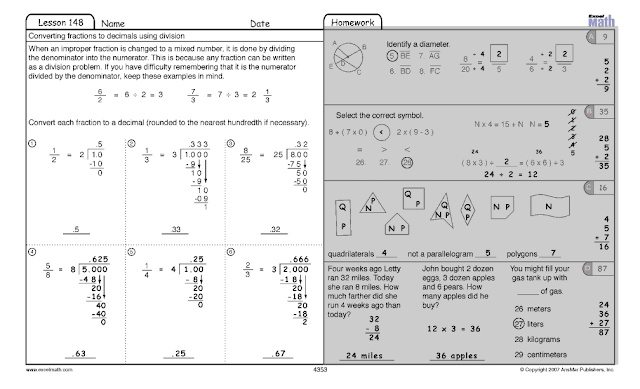## Wednesday, April 25, 2012

### Recognizing Equivalent Representations of Fractions: Music

Fractions that refer to the same portion of a group of items or the same part of a whole are called equivalent fractions. (Read our April 11 and April 16 blog posts for more on the topic of equivalent fractions.)

So one-fourth can be notated as a fraction in these ways, among others:These two fractions equal the same thing so they are equivalent fractions.

In Excel Math, students learn to recognize equivalent fractions. In addition, they learn to convert fractions to decimals. Some of our math students are also learning to play musical instruments. In fact, research shows that students who study music often do better in math than students without a music background. Since music involves quite a bit of math and triggers some of the same brain functions as are triggered when learning math concepts, that makes sense.

Today let's look at equivalent fractions using musical notes and rests. Then we'll take a look at converting fractions to decimals. This is a quarter note. A quarter note gets one beat:Here's a whole note. A whole note gets 4 beats:

Therefore, four quarter notes (which get one beat each) equal one whole note (which gets four beats):
A quarter note equals one-quarter of a whole note. So if a whole note gets four beats, a quarter note gets one beat or one-fourth of the whole, which can be represented as a fraction:
This is a quarter rest. A quarter rest gets one beat of silence:

Here's a whole rest. A whole rest gets 4 beats of silence:

So a quarter rest equals one-quarter of a whole rest. If a whole rest gets 4 beats of silence, a quarter rest gets 1 beat of rest or one-fourth of the whole:

In Excel Math, students learn how to convert fractions to decimals. In our first example above, one-fourth can be written as fraction, but it can also be written as a decimal:

When a fraction is changed to a decimal, the denominator (the number down below the line) is divided into the numerator. So any fraction can be written as a division problem:

In Excel Math, students also learn how to convert improper fractions to mixed numbers using division. When an improper fraction is changed to a mixed number, it is done by dividing the denominator into the numerator. This is because any fraction can be written as a division problem. If your students have difficulty remembering that it is the numerator divided by the denominator, remind them to keep these examples in mind:

We've given you a free Excel Math worksheet you can print out and use with your students to help them remember to use division to convert fractions to decimals. Click here for the PDF file you can download. The first three problems are done for your students so they can see how to get started. You may want to work them together as a class. Then have one student show the others how to solve number 4. Do the same for 5 and 6.Click here for this free math worksheet you can print out and use with your students.
Excel Math Grade 4 Lesson 148 Student Worksheet

The answer sheet for these problems is below. (However, since Excel Math uses a unique spiraling strategy, you will need to teach multiple lessons sequentially within each grade level in order to get the concepts into your students' long-term memory.) Visit our web store to order these Student Lesson Sheets (\$11.00 per student for 155 lesson sheets plus tests) and a Teacher Edition set of 155 Lessons with brainteasers, teaching suggestions, the answer key, and reproducible manipulatives. (Teacher Edition for each age level is just \$30.00.) Excel Math is available for Kindergarten through Grade 6.

This is what one teacher had to say about how Excel Math lessons work in the classroom:
“We are using your Excel Math in our primary grades. We love it! By using it across grade levels, it allows us to differentiate by grouping out students according to their ability, not just their grade. It also allows us to keep the higher students challenged, and grade level students right on track while allowing plenty of review for the lower students. Excel Math is an excellent program for any school.” – 2nd Grade Teacher from Aptos, California
Here are the answers to the worksheet above:Excel Math Grade 4 Lesson 148 Student Worksheet with Answers